Theory and Modern Applications

# Comparison and validation of integer and fractional order ultracapacitor models

## Abstract

In this article, the modeling of the ultracapacitor using different models of capacity part is shown. Two fractional order models are compared with the integer model of traditional capacitor. The identification was made using the diagram matching technique. Next, the derivation of time domain response of the ultracapacitor and system with the ultracapacitor are presented. The results of frequency domain identification were used to validate the response of the ultracapacitor in time domain. All theoretical results are compared with the response of the physical system with the ultracapacitor.

## Introduction

Ultracapacitors (aka supercapacitors) are electrical devices which are used to store energy and offer high power density that is not possible to achieve with traditional capacitors. Nowadays, ultracapacitors have many industrial applications and are used wherever a high current in a short time is needed. Thanks to a very complicated internal structure, they are able to store or yield a lot of energy in a short period of time. Many researchers started building a more or less complicated model to explain the capability of ultracapacitors. Numerous articles have presented the RC model (e.g., ), which is particularly accurate for low frequencies. Some authors describe ultracapacitors by the RC transmission line . Also, the dynamic behavior of ultracapacitors has been modeled using the technique based on impedance spectroscopy in, e.g., . In the papers,  a very efficient approach using fractional order calculus was presented and in [10, 11] ultracapacitor frequency domain modeling was introduced. In this article, modeling using three different models of ultracapacitors are compared. Two of them are fractional order. We validate the identified models from frequency domain  with the step response of this model in time domain. The time domain responses of the ultracapacitor and a system with the ultracapacitor are calculated. All theoretical results are compared with the results achieved from a physical system.

## Fractional order differential calculus introduction

Fractional order differential calculus is only a generalization of integer order integral and differential calculus to real or even complex order. This idea has first been mentioned at the end of seventeenth century. There exist two (in fact three) main definitions of the fractional order integrals and derivatives: Riemann-Liouville (and Caputo) and Grünwald-Letnikov . In this article, the Riemann-Liouville definition of the fractional order difference is used.

### Definition of fractional order differ-integral

In this article, the following definition of the fractional derivative will be used:

Definition 1. Riemann-Liouville (R-L) definition of fractional order differ-integralwhere t , a , t > a and α is a fractional order of the differ-integral of the function f(t). For α > 0 m - 1 < α m, m and for α 0 m = 0.

When α > 0 the result of this function is equivalent to the fractional order derivative, for α < 0 to fractional order integral and for α = 0 to the function itself. This is why the above definition is called a differ-integral.

The Laplace transformation of the fractional order differ-integral in Riemann-Liouville form is given as follows: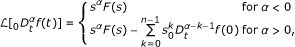(1)

whereIt is easy to see that the Laplace transformation of the Riemann-Liouville definition possesses the fractional order derivatives of initial conditions. Despite the difficulty in finding the physical meaning of these parameters, using the R-L definition to model the ultracapacitor is feasible, because the model presented in this article is based on the fractional order transfer function, where initial conditions are equal to zero.

### Fractional order integrator

Let us assume the following transfer function of a fractional order integrator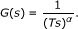(2)

The spectral transfer function of (2) is obtained for s = as follows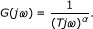(3)

The magnitude of the transfer function is given as follows(4)

which yields(5)

The phase properties are obtained from the following:(6)

The Bode diagram of the fractional order integrator for different values of α is presented in Figure 1.

## Frequency domain models of ultracapacitor

Ultracapacitors are large capacity and power density electrical energy storage devices. This large capacity is the effect of a very complicated internal structure. This structure also has a significant impact on the dynamic behavior of the ultracapacitor. Many authors use different RC models to describe the performance of the ultracapacitors but these models are correct only for a limited range of frequencies. A more effective approach is based on using the fractional order model which gives highly accurate results of modeling over a wider range of frequencies. In this article, we would like to show the advances of using fractional order model for ultracapacitor modeling.

### Fractional order ultracapacitor model

The transfer function of the modeled system in Figure 2 is defined as: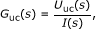where U uc(s) is a Laplace transform of the capacitor voltage and I(s) is a Laplace transform of the capacitor current. In case of modeling capacity part of ultracapacitor we use three models presented below: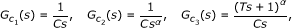where α is the model order, C is the capacity of ultracapacitor and T + is the parameter responsible for capacity decrease with frequency . The first model is the integer order model of the ideal ultracapacitor. The other two are the fractional ones. The whole transfer function of ultracapacitor presented in Figure 2 is: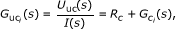(7)

where R c is the resistance of the ultracapacitor and i is the model index (i = 1, 2, or 3).

## Frequency domain identification of ultracapacitors parameters

The modeling of the ultracapacitor presented in this article is based on the diagram matching. For modeling we used two experimental systems. The first one is to examine high-capacity ultracapacitors and the second one to examine low-capacity ultracapacitors.

### Modeling high-capacity ultracapacitor

To model a high-capacity ultracapacitor the experimental setup contained high-capacity ultracapacitor connected to the DS1104 Control Card by the electronic interface based on the MOSFET power converter. The research was focused on the Maxwell® ultracapacitor of nominal capacity 1500F/2.7V (BCAP1500) and 3000F/2.7V (BCAP3000).

The identification was based on Bode diagram matching. A Bode diagram of the models was tuned to the diagram of the ultracapacitor which was determined from measurements. As a result of this research, we obtained the parameters of the models.

Using the experimental setup to examine high-capacity ultracapacitors, we were able to use high current to charge and discharge ultracapacitors (current values of more than 100 A were used). Additionally, using this system we were also able to show the physical step response of the ultracapacitor.

### Modeling low-capacity ultracapacitor

To present the step response of the system with an ultracapacitor and to examine the low-capacity ultracapacitor, the following experimental setup was built (Figure 3).

This setup is composed of the operational amplifier OPA544, matching resistor 180Ω and ultracapacitors produced by Panasonic® of nominal capacity 0.047 F, 0.1 F, 0.33 F. The high current operational amplifier OPA 544 works in the voltage follower configuration.

Also in this case, the modeling of the ultracapacitor was based on Bode diagram matching and ultracapacitor models shown above.

### Results of experiments

The ultracapacitor is an electrolytic capacitor and it can accept only positive voltages. In the case of using this type of setup with a current converter, to model high-capacity ultracapacitor, the input signal was a current sine wave. The ultracapacitors had an initial voltage depending on a signal frequency (u 0). Capacitor voltage in this case was equal to u c (t) = u 0 + A c (ω)sin(ωt + φ u ), and input (capacitor) current was i(t) = A i (ω)sin(ωt + φ i ).

In the case of a system with a voltage follower, the configuration used a voltage input signal with a constant component u(t) = 2 + sin(ωt). Capacitor voltage (in steady state) in this case was equal to u c (t) = 2 + A c (ω)sin(ωt + φ u ) and capacitor current was i(t) = A i (ω)sin(ωt + φ i ).

The Bode diagram was obtained from the following relations:where A c (ω) is the magnitude of ultracapacitor voltage and A i (ω) is the magnitude of current flowing through ultracapacitor for used frequency. The φ i (ω) and φ u (ω) are the phase shifts between input system voltage and current flowing through ultracapacitor and voltage of the ultracapacitor, respectively.

The parameters obtained as a result of parameters identification of ultracapacitor of nominal capacity 1500 F using different models by diagrams matching are presented in Table 1. In Figures 4, 5, and 6, the results of identification in frequency domain are presented. It is easy to see that the fractional order model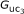has the best match to the measured data (see Figure 7). This diagram shows its fit in the fairly wide range of frequencies (however not for all frequencies used). Even though, all three models gave satisfactory results in frequency domain for high-capacity ultracapacitors, the results of identification for low-capacity ultracapacitor 0.33F, presented in Figures 8, 9, and 10 are only acceptable for themodel (see Figure 11).

For the model, we had also compared the identified parameters with the physical parameters of low capacity ultracapacitors measured directly from ultracapacitors by means of step response. The results are shown in Table 2.

The result of identification using fractional order modelgave very good parameters which means that this model can very precisely model the ultracapacitors. Their parameters are equivalent to these obtained from real ultracapacitor by different methods, e.g., step response. The approach presented in this section is in fact analogous to the impedance spectroscopy. However in our case, we rather use Bode plot frequency response as opposed to Nyquist diagram.

## Validation of time domain response of ultracapacitor

In this section, the validation of models with identified parameters in time domain are presented.

### Time domain response derivation

The time response of a traditional capacitor for the step input of current equal to I is well known to be:(8)

The same time response of capacitor with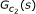capacity model has the following form:(9)

Calculation of this time response of ultracapacitor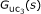model is however more complicated.

Lemma 1. The time domain step response of the ultracapacitor itself is as follows:(10)

where theis the confluent hypergeometric function.

Proof. To calculate the step response of ultracapacitor withcapacity model is necessary to find the inverse Laplace transformation presented below: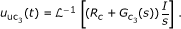(11)

The hardest task in this calculus is finding the inverse Laplace transformation of unit step of the capacity part:(12)

which can be rewritten as: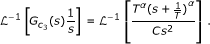(13)

Using the theorem of the complex shift of the Laplace transformation, we obtain:(14)

To solve the inverse Laplace transformation from (14), the following formula is used: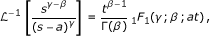(15)

where γ, β +, and the 1 F 1(γ;β ; at) is the confluent hypergeometric function defined as :and (b) n , (c) n are the Pochhammer symbols defined by:Using (15) to (14) we calculate the step response of the ultracapacitor in time domain: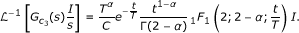(16)

Analytically, we can also calculate the response of the ultracapacitor with the linear function of current (i(t) = It, where I = const) as an input signal: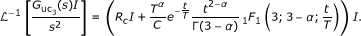(17)

### Step response of the system with ultracapacitor

The RC quadripole we defined as follows:for i = { 1, 2, 3}.

The step response of RC quadripole with the ultracapacitor model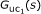is: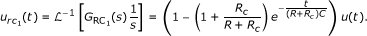(18)

The step response of RC quadripole with the ultracapacitor modelis: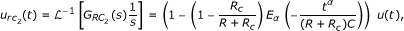(19)

whereis the one-parameter representation of the Mittag-Leffler function below.

Definition 2.The one-parameter representation of the Mittag-Leffler function can be defined in terms of a power series  written as:where α > 0.

Calculation of the step response of RC quadripole with the ultracapacitor modelfor arbitrary α is not easy. Therefore, we present the resulting step response of this model for α = 0.5. The experiments performed revealed that for low-capacity ultracapacitors α is close enough to 0.5 to provide correct modeling of ultracapacitor dynamics.

Lemma 2. The step response of the system with the ultracapacitor for α = 0.5 is given by the following equation: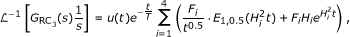(20)

whereandis a two-parameter Mittag-Leffler function. Parameters C, R c , T are the parameters of the ultracapacitormodel and R is the resistance of RC quadripole's resistor.

Proof. To calculate the step response of the system with the ultracapacitor in the time domain for α = 0.5 it is necessary to find the inverse Laplace transformation of the following transfer function: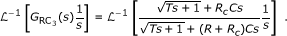(21)

The above equation can be rewritten as:(22)

where B = R c C and A = (R + R c )C.

As a result of the applied theorem of the complex shift of the Laplace transformation, we obtain:(23)

Let us define the auxiliary variable: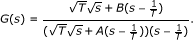(24)

Using the auxiliary complex variable w = s 0.5 we have: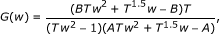(25)

After decomposition of G(w) into the partial fractions and inverse Laplace transform, back in the complex variable s we find:By linearity of the Laplace transformation, the goal of finding the inverse Laplace transformation was decomposed to four identical problems.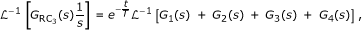(26)

where G 1(s), G 2(s), G 3(s), and G 4(s) are the individual partial fraction. All of them we can transform using new parameters to the presented form: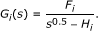Using the following equation for decomposition of rational function to partial fraction:(27)

where, we obtained:The inverse Laplace transformation can be calculated using the following equation:(28)

where function E 1,κ+1(at) is a two-parameter Mittag-Leffler function , defined as:for α, β > 0. Finally, from (26), (27), and (28) we obtained the step response of the system with the ultracapacitor.

### Results of identification in time domain

To validate the model with the parameters obtained in frequency domain identification the step responses of all the models were derived. The results of this validation are presented in Figures 12, 13, and 14. These figures show the step responses of ultracapacitor with nominal capacity 1500 F for current input signal equal to 100 A.

These figures present that fractional order modelgives the best result of the ultracapacitor modeling in frequency and also in time domain.

In Figure 15, it is shown that step response of RC quadripole with ultracapacitor 0.33 F using parameters achieved in frequency domain identification for modelyields also very good matching.

## Conclusions and future works

### Conclusions

In the article, the results of frequency and time domain modeling of ultracapacitors, using three different models, have been presented. The best description of the ultracapacitor dynamic was achieved using fractional model in the formwhich describes the dynamics of the ultracapacitor over a reasonable range of frequencies. This model of the ultracapacitor can be used in either time or frequency domains. The model proposed provides good results of modeling in time domain, in comparison with the other models considered. However, to cover even wider range of frequencies the intrinsic nonlinearities of the ultracapacitors have to be taken into account resulting in either a time-varying or nonlinear model.

Modelis also better for modeling ultracapacitors thenfractional order model from the point of view of physical interpretation. Because the model corresponds to the physical ultracapacitor we use the integer order in the denominator, otherwise if the order of ultracapacitor is in the range 0 < α < 1 then the capacity of such a device tends to infinity with time, which is not possible in the case of physical systems .

### Future works

The model obtained with the technique presented may form the foundation for establishing the control design procedures. The scope of future works will also deal with building the state space model of the ultracapacitor which will be composed of the part responsible for the integer order capacitor and the fractional order part responsible for a better description of the behavior and all the specific phenomena of the ultracapacitors.

## References

1. Bertrand N, Briatt O, Vinassa J-M, Sabatier J, El Brouji H: Porous electrode theory for ultracapacitor modelling and experimental validation. Proceedings of IEEE Vehicle Power and Propulsion Conference, VPPC'08, Harbin, China,3–5September 2008

2. Buller S, Karden E, Kok D, De Doncker RW: Modeling the dynamic behavior of supercapacitors using impedance spectroscopy. IEEE Trans Industry Appl 2002,38(6):1622-1626. 10.1109/TIA.2002.804762

3. El Brouji H, Vinassa J-M, Briat O, Bertrand N, Woirgard E: Ultracapacitors self discharge modelling using a physical description of porous electrode impedance. Proceedings of IEEE Vehicle Power and Propulsion Conference, VPPC'08, Harbin (China),3–5September, 2008

4. Lajnef W, Vinassa J-M, Briat O, Azzopardi S, Woirgard E: Characterization methods and modelling of ultracapacitors for use as peak power sources. J Power Sour 2008, 168:, 553-560.

5. Shi L, Crow ML: Comparison of ultracapacitor electric circuit models. Proceedings of IEEE Power and Energy Society General Meeting, Pittsburgh, PA, USA 2008, 1-6.

6. Belhachemi F, Rael S, Davat B: A physical based model of power electric double-layer supercapacitors. Proceedings of IEEE Industry Applications Conference, 35th IAS Annual Meeting 2000, 5: 3069-3076.

7. Quintana JJ, Ramos A, Nuez I: Identification of the fractional impedance of ultracapacitors. Proceedings of the 2nd IFAC Workshop on Fractional Differentiation and its Applications, IFAC FDA06, Porto, Portugal, 19-21 July 2006

8. Riu D, Retière N, Linzen D: Half-order modelling of supercapacitors. Proceedings of IEEE Industry Applications Conference, 39th IAS Annual Meeting, vol. 4, Seattle, WA, USA, pp 2550-2554, Oct 2004

9. Westerlund S, Ekstam L: Capacitor theory. IEEE Trans Dielectr Electr Insulation 1994, 1: 826-839. 10.1109/94.326654

10. Dzieliński A, Sierociuk D: Ultracapacitor modelling and control using discrete fractional order state-space models and fractional Kalman filter. Proceedings of European Control Confrence, ECC2007, Kos, Greece, pp 2916-2922,2–5July 2007

11. Dzieliński A, Sierociuk D: Ultracapacitor modelling and control using discrete fractional order state-space model. Acta Montan Slovaca 2008,13(1):136-145.

12. Dzieliński A, Sierociuk D, Sarwas G: Ultracapacitor parameters identification based on fractional order model. Proceedings of European Control Conference 2009, Budapest, Hungary, 23-26 August, 2009

13. Podlubny I: Fractional Differential Equations. Academic Press, San Diego-Boston-New York-London-Tokyo-Toronto; 1999.

14. Wang J, Li Y: Frequency domain analysis and applications for fractional-order control systems. J Phys Conf Ser 2005, 13: 268-273.

15. Magin RL: Fractional calculus in bioengineering. Critical Rev Biomed Eng 2004,32(1):1-104. 10.1615/CritRevBiomedEng.v32.10

16. Kilbas AA, Saigo M, Saxena RK: Generalized Mittag-Leffler function and generalized fractional calculus operators. Integral Transform Special Funct 2004,15(1):31-49. 10.1080/10652460310001600717

## Acknowledgements

This work was partially supported by the Polish Ministry of Science and Higher Education Grant number 4125/B/T02/2009/36 and the European Union in the framework of European Social Fund through the Warsaw University of Technology Development Programme (by Centre for Advanced Studies WUT).

## Author information

Authors

### Corresponding author

Correspondence to Andrzej Dzieliński.

### Competing interests

The authors declare that they have no competing interests.

### Authors' contributions

AD conceived of the study, performed its theoretical analyses, and participated in its design and coordination. GS carried out the experiments and the derivation of step response formula. DS designed the experimental setup of the study and performed the analyses.

## Authors’ original submitted files for images

Below are the links to the authors’ original submitted files for images.

## Rights and permissions

Reprints and Permissions

Dzieliński, A., Sarwas, G. & Sierociuk, D. Comparison and validation of integer and fractional order ultracapacitor models. Adv Differ Equ 2011, 11 (2011). https://doi.org/10.1186/1687-1847-2011-11